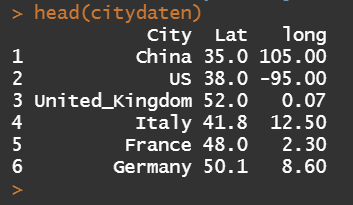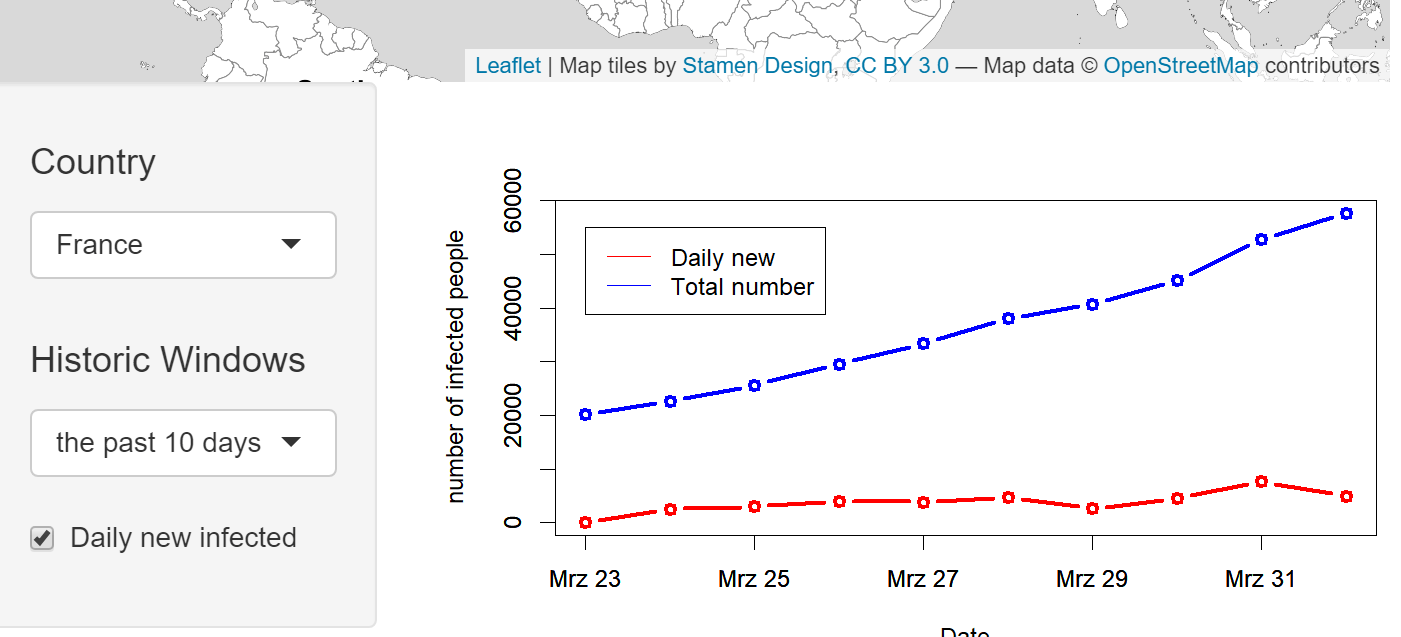# Hands-on: How to build an interactive map in R-Shiny: An example for the COVID-19 Dashboard

April 15, 2020
By

Want to share your content on R-bloggers? click here if you have a blog, or here if you don't.

It has been two years since I started to develop various interactive web applications by using R-Shiny packages. Due to Coronavirus disease (COVID-19) at this moment, I spent some time at home on preparing a simple COVID-19 related Dashboard. To share my experiences and consideration, especially those about how to create interactive maps, the following details has been discussed.

Data Source

Dataset (data/key-countries-pivoted.csv) from Github (https://github.com/datasets/covid-19), which contains the data of daily confirmed cases of COVID-19 since 22th Jan 2020 from the eight most rapidly-spread countries (China, USA, United Kingdom, Italy, France, Germany, Spain and Iran), has been used. An example of overview of the dataset is given as follows:In addition, the geographic coordinates (Latitude and Longitude) of the centroids of these countries have been collected for placing charts in the leaflet map.R Shiny: Introduction

Shiny application consists of two components, a user interface object and a server function. In user interface object, you are able to create dynamic dashboard interface, while the server function contains code provides an interactive connection between objects used for input and output. The UI object and server function will be further listed, respectively.

R library

We use the R library leaflet, which is a Javascript Library and allows users to create and customize interactive maps. For more details, we refer users to the link: https://cran.r-project.org/web/packages/leaflet/index.html

``` library(shiny)
library(leaflet.minicharts)
library(leaflet)```

Shiny-UI

The user Interface is constructed as follows, where two blocks are included. The upper block shows the worldwide COVID-19 distribution and the total number of the confirmed cases of each country. The amount of cases is labelled separately and those countries with more than 500,000 cases are marked as red, otherwise black. Note that the function leafletOutput() is used for turning leaflet map object into an interface output.In the lower block, we are able to visualize the time dependent development of confirmed cases for a selected country and time history.  The classical layout sideBarLayout, which consists of a sidebar (sidebarPanel()) and the main area (mainPanel()), is used. Moreover, a checkbox is provided to select whether plotting the daily new confirmed cases is desired.The R code for UI is given as follows:

``` ui<- fluidPage(
#Assign Dasbhoard title
titlePanel("COVID19 Analytics"),

# Start:  the First Block
# Sliderinput: select from the date between 01.20.2020
# and 01.04.2020
sliderInput(inputId = "date", "Date:", min =
as.Date("2020-01-20"), max = as.Date("2020-04-01"),
value = as.Date("2020-03-01"), width = "600px"),

# plot leaflet object (map)
leafletOutput(outputId = "distPlot", width = "700px",
height = "300px"),
#End:  the First Block

#Start: the second Block
sidebarLayout(

#Sidebar Panel: the selected country, history and
#whether to plot daily new confirmed cases.
sidebarPanel(
selectInput("selectedcountry", h4("Country"), choices
=list("China","US","United_Kingdom","Italy","France",
"Germany", "Spain"), selected = "US"),
selectInput("selectedhistoricwindow", h4("History"),
choices = list("the past 10 days", "the past 20
days"), selected = "the past 10 days"),
checkboxInput("dailynew", "Daily new infected",
value = TRUE),
width = 3
),

#Main Panel: plot the selected values
mainPanel (
plotOutput(outputId = "Plotcountry", width = "500px",
height = "300px")
)
),
#End: the second Block
)```

Shiny-Server

Input and output objects are connected in the Server function. Note that, the input arguments are stored in a list-like object and each input argument is identified under its unique name, for example the sliderInput is named after “date”.

```server <- function(input, output){

#Assign output\$distPlot with renderLeaflet object
output\$distPlot <- renderLeaflet({

# row index of the selected date (from input\$date)
rowindex = which(as.Date(as.character(daten\$Date),
"%d.%m.%Y") ==input\$date)

# initialise the leaflet object
basemap= leaflet()  %>%
options = providerTileOptions(noWrap = TRUE))

# assign the chart colors for each country, where those
# countries with more than 500,000 cases are marked
# as red, otherwise black
chartcolors = rep("black",7)
stresscountries
= which(as.numeric(daten[rowindex,c(2:8)])>50000)
chartcolors[stresscountries]
= rep("red", length(stresscountries))

# add chart for each country according to the number of
# confirmed cases to selected date
# and the above assigned colors
basemap %>%
citydaten\$long, citydaten\$Lat,
chartdata = as.numeric(daten[rowindex,c(2:8)]),
showLabels = TRUE,
fillColor = chartcolors,
labelMinSize = 5,
width = 45,
transitionTime = 1
)
})

#Assign output\$Plotcountry with renderPlot object
output\$Plotcountry <- renderPlot({

#the selected country
chosencountry = input\$selectedcountry

#assign actual date
today = as.Date("2020/04/02")

#size of the selected historic window
chosenwindow = input\$selectedhistoricwindow
if (chosenwindow == "the past 10 days")
{pastdays = 10}
if (chosenwindow  == "the past 20 days")
{pastdays = 20}

#assign the dates of the selected historic window
startday = today-pastdays-1
daten\$Date=as.Date(as.character(daten\$Date),"%d.%m.%Y")
selecteddata
= daten[(daten\$Date>startday)&(daten\$Date<(today+1)),
c("Date",chosencountry)]

#assign the upperbound of the y-aches (maximum+100)
upperboundylim = max(selecteddata[,2])+100

#the case if the daily new confirmed cases are also
#plotted
if (input\$dailynew == TRUE){

plot(selecteddata\$Date, selecteddata[,2], type = "b",
col = "blue", xlab = "Date",
ylab = "number of infected people", lwd = 3,
ylim = c(0, upperboundylim))
par(new = TRUE)
plot(selecteddata\$Date, c(0, diff(selecteddata[,2])),
type = "b", col = "red", xlab = "", ylab =
"", lwd = 3,ylim = c(0,upperboundylim))

legend(selecteddata\$Date, upperboundylim*0.95,
legend=c("Daily new", "Total number"),
col=c("red", "blue"), lty = c(1,1), cex=1)
}

#the case if the daily new confirmed cases are
#not plotted
if (input\$dailynew == FALSE){

plot(selecteddata\$Date, selecteddata[,2], type = "b",
col = "blue", xlab = "Date",
ylab = "number of infected people", lwd = 3,
ylim = c(0, upperboundylim))
par(new = TRUE)

legend(selecteddata\$Date, upperboundylim*0.95,
legend=c("Total number"), col=c("blue"),
lty = c(1), cex=1)
}

})

} ```

Shiny-UI

At the end, we create a complelte application by using the shinyApp function.

` shinyApp(ui = ui, server = server)`

The Dashboard is deployed under the following URL: https://sangmeng.shinyapps.io/COVID19/

R-bloggers.com offers daily e-mail updates about R news and tutorials about learning R and many other topics. Click here if you're looking to post or find an R/data-science job.
Want to share your content on R-bloggers? click here if you have a blog, or here if you don't.